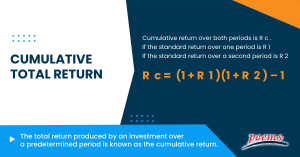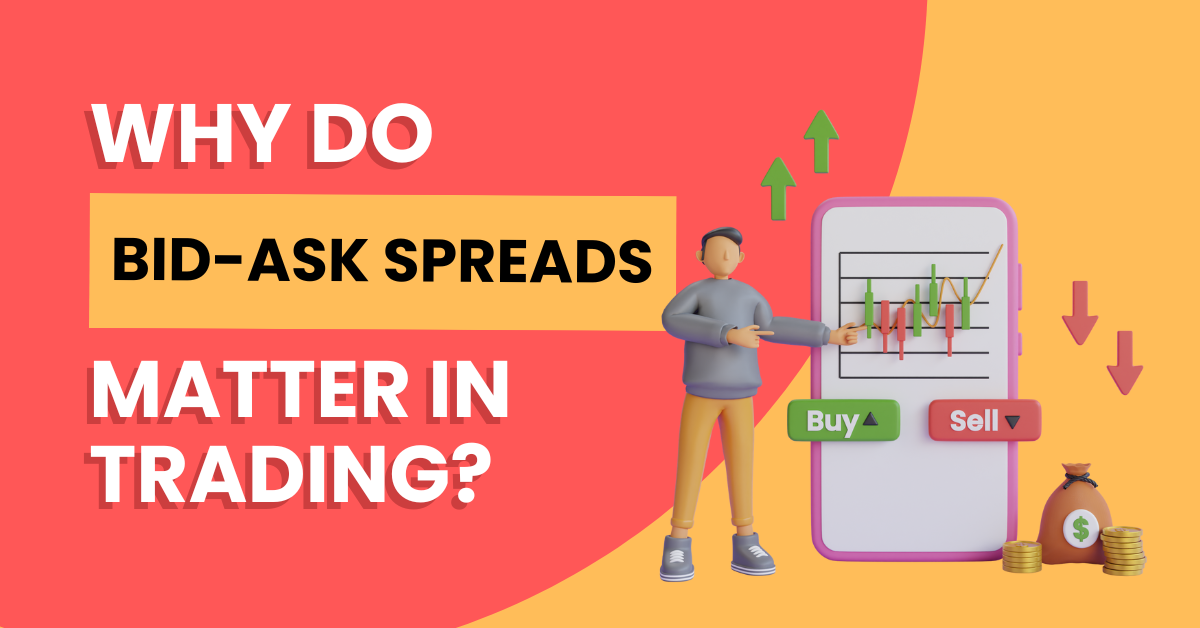﻿ Cumulative total return: What is it, Formula, calculate, example, FAQ

# Cumulative total return

## Cumulative total return

If investments didn’t yield returns, why would anyone take the chance? Returns can be examined in a variety of ways.

Comparing the investment’s final value to its initial primary value, cumulative returns examine its return over a longer time frame. It serves largely as a method of analyzing an investment’s overall performance over time.## What is cumulative total return?

The total return produced by an investment over a predetermined period is known as the cumulative return. It is the total gain or loss on investment throughout time, regardless of the time involved.

The overall change in the investment’s price over a predetermined period is the cumulative return; this is a total return, not an annualized return. Reinvested dividends or capital gains impact an investment’s cumulative return.

This kind of return takes profits and losses from the period into account and bases the final total on the change in value from the start to the end of the same period. A cumulative return is typically shown as a percentage rather than a monetary value.

## Understanding cumulative total return

Calculating the profit or loss over the purchase price makes it simple to determine the cumulative return of an asset that does not pay interest or dividends. That can be effective with assets like growth stocks and precious metals that don’t pay dividends. The cumulative return can be computed using the raw closing price.

The cumulative return of all assets can be easily calculated using the adjusted closing price. This covers investments like dividend-paying equities and bonds that pay interest. The adjusted closing price considers the impact of interest, dividends, stock splits, and other price changes on the asset. Therefore, it is possible to calculate the cumulative return by utilizing the security’s first adjusted closing price as the purchase price.

## The formula for cumulative total return

The cumulative return over both periods is R c if the standard return over one period is R1 and R2 if the standard return over a second period,

Rc is equal to (1 + R1)(1 + R2) – 1

The total return is another name for the cumulative return.

Although there are ways to calculate additive cumulative returns, the cumulative return, as described here, is not additive across sub-portfolios.

## How do you calculate cumulative total return?

When the cumulative rate of return is added to this equation, it becomes: (1 + RA) n = 1 + RC. Where n is the number of years taken into account in the computation of RC, RC is the cumulative rate of return, and RA is the annualized rate of return.

Using the above example, we can deduce that n = 5 and RC = 0.4. The result of fiddling with the equation is RA = [(1 + RC) (1/n)]. -1. Calculators for these mathematical issues can be found online; in this example, the yearly rate of return is 0.6961, or 6.96 percent. This is less than the inaccurate 8 percent that you would have estimated using basic math.

Using the annual compounded return as a starting point, you can compare the cumulative return with other potential investments. This can aid in your decision-making over whether to keep your money where it is currently invested or move it to a location with higher returns.

## Example of cumulative total return

To better understand cumulative total return, let’s look at the following example:

Suppose you paid \$10,000 for 100 shares of stock A five years ago. Your shares of stock are now worth \$14,000, giving you a \$4,000 increase over five years. Cumulative return is calculated as (\$4,000 gain) / (\$10,000 original investment) = 0.4 or 40%.

Your investment has generated a total return of 40% over the past five years. To determine which assets outperformed the others, you may compare this figure to the cumulative returns on other investments over five years. However, unless you have a straightforward loan that doesn’t compound interest, you can’t divide this number by five to achieve an annual compound return of (0.4 / 5) = 0.08, or 8 percent.

The investor will use the following calculation to determine the total return rate (which is required to get the annualized return): Starting value = (ending value – beginning value)

Most investors consider an average annual return of 10% or above to be a decent ROI for long-term stock market investments.

There are a few key differences between annualized returns and cumulative returns.

• Annualized return is calculated by taking the average return over a specified period, typically one year. On the other hand, cumulative return sums up the total return over a specified period.
• Additionally, an annualized return takes into account the time value of money, meaning that it accounts for the fact that money today is worth more than money in the future. Cumulative return does not take this into account.
• Finally, the annualized return is a more accurate measure of investment performance than cumulative return since it accounts for the effects of compounding. Cumulative return does not account for compounding and can be misleading.

The simple cumulative daily return is determined by multiplying the daily percentage change by the cumulative factor.

The cumulative total return is an investment’s total return over time. This includes both the initial investment and any subsequent reinvestments. It is a measure of the overall performance of an investment.

The cumulative total return is significant because it provides a more accurate picture of an investment’s true return. It takes into account not only the initial investment but also any reinvestments that have been made. This makes it a more accurate measure of the investment’s overall performance.

The cumulative total return is a useful tool for investors because it allows them to compare the performance of different investments. It is also helpful in determining an investment portfolio’s overall risk and return.

## Category

### Read the Latest Market Journal#### Top traded counters in August 2023

Published on Sep 19, 2023 125#### Weekly Updates 18/9/23 – 22/9/23

Published on Sep 18, 2023 20

This weekly update is designed to help you stay informed and relate economic and company...#### The Merits of Dollar Cost Averaging

Published on Sep 15, 2023 39

Have you ever seen your colleagues, friends or family members on the phone with their...#### Singapore Market: Buy the Dip or Dollar-Cost Averaging?

Published on Sep 14, 2023 46

To the uninitiated, investing in the stock market can be deemed exhilarating and challenging. The...#### What are covered calls and why are they so popular?

Published on Sep 12, 2023 333Published on Sep 11, 2023 30#### Weekly Updates 11/9/23 – 15/9/23

Published on Sep 11, 2023 13

This weekly update is designed to help you stay informed and relate economic and company...#### Exploring the Rise and Fall of the Japanese Yen (JPY) Through Time

Published on Sep 5, 2023 79

The Japanese Yen (JPY) has long been considered a safe-haven currency due to Japan’s strong...